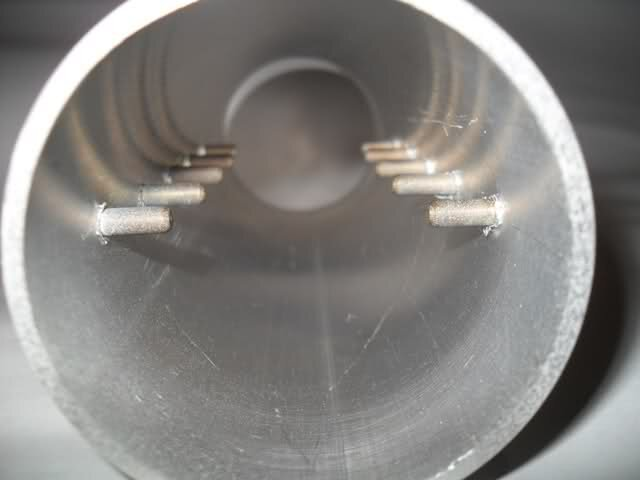# waveguide calculating

#### dreambox59

##### Active SatelliteGuys Member
i am studying av-comm polarizer and "polopipe" (from brazil) (device for convert circular to linear)
i have found some calculation about wavelenght in pipe but i have two variant of the same equation :

LG = L / (racine (1 - ( L / (2.d))^ 2))
LG = L / (racine (1 - ( L / (1.6406.d))^ 2))

lg = wavelenght in pipe
L=wavelenght in air
d=pipe diameter

in the second way 1.64.06 matches with polopipe described on south american forums
first way is classical value found in mathmatical topics

does someone knows this subject and can gives me advice•k.r. and martin-f